### Home > MC1 > Chapter 7 > Lesson 7.3.2 > Problem7-112

7-112.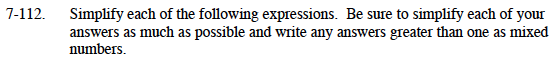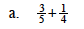Make the fractions have the same denominator.

$\left ( \frac{3}{5} \right )\left ( \frac{4}{4} \right )=\frac{12}{20}\ \ \ \ \ \ \left ( \frac{1}{4} \right )\left ( \frac{5}{5} \right )= \frac{5}{20}$

$\frac{12}{20}+\frac{5}{20}=\frac{17}{20}$

$\frac{17}{20}$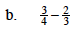You will follow the same steps as in part (a), except that you will subtract two-thirds from three-fourths, rather than adding them.

$\frac{1}{12}$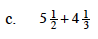You can either convert these mixed numbers to fractions greater than one before adding, or you can add their parts. Remember that your answer should be expressed as a mixed number.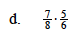To multiply fractions, you multiply the numerators by one another to find the new numerator. The same process is repeated for finding the new denominator.